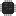# Piping in AWK

December 10, 2022

AWK is often used as part of a pipeline of shell commands. However, have you ever opened a pipe from within AWK?

A few weeks ago I was trying to do some quick alignment of attributes in XML files. For example, turning

``<SomeTag a="aa" b="bbbbbb" c="cccccc"><SomeTag a="aaaaa" b="bbbbbbbbbbbbbb" c="cc"><SomeTag a="a" b="b" c="c"><SomeOtherTag a="aa" b="bbbbbb"><SomeOtherTag a="aaaaa" b="bb">``

into

``<SomeTag  a="aa"     b="bbbbbb"          c="cccccc"><SomeTag  a="aaaaa"  b="bbbbbbbbbbbbbb"  c="cc"><SomeTag  a="a"      b="b"               c="c"><SomeOtherTag  a="aa"     b="bbbbbb"><SomeOtherTag  a="aaaaa"  b="bb">``

to make it easier to quickly compare the values for each attribute.

I thought it might be easy to write a quick solution with AWK. However, for each chunk of tags, I would prefer if I could utilize `column -t` and not have to reimplement the alignment. Searching for a `|` in the awk(1p) man page quickly revealed a feature of AWK that I had not been aware of before:

Both print and printf statements shall write to standard output by default. The output shall be written to the location specified by outputredirection_ if one is supplied, as follows:

``> expression>> expression| expression``

In all cases, the `expression` shall be evaluated to produce a string that is used as a pathname into which to write (for `>` or `>>`) or as a command to be executed (for `|`). Using the first two forms […].

The third form shall write output onto a stream piped to the input of a command. The stream shall be created if no stream is currently open with the value of `expression` as its command name. The stream created shall be equivalent to one created by a call to the `popen()` function defined in the System Interfaces volume of POSIX.1‐2017 with the value of `expression` as the `command` argument and a value of w as the mode argument. As long as the stream remains open, subsequent calls in which expression evaluates to the same string value shall write output to the existing stream. The stream shall remain open until the close function (see Input/Output and General Functions) is called with an expression that evaluates to the same string value. At that time, the stream shall be closed as if by a call to the `pclose()` function defined in the System Interfaces volume of POSIX.1‐2017.

To summarize, any print statement can be followed by a `|` and a string. That string will be executed as a shell command with the printed value sent to `stdin`. This pipe will remain open until either `close()` is called with an exactly identical string or the awk program is finished. While it is open, one can continue adding more data to `stdin` by having more prints piped to the exact same command string.

So for each line of a chunk we can pipe the line to `column` and whenever we leave the chunk we close the file so we get a new instance of `column` for the next chunk:

``BEGIN { cmd="column -t" }/^<.+>\$/ { print | cmd; next }{ close(cmd); print }``

Running it on our example above yields exactly the desired result above.

For this year’s Advent of Code I have mostly been using AWK and on the very first day I realized I could make use of this feature. Keep in mind, there will be mild spoilers for this year’s event if you haven’t solved them yet, specifically for day 1 and 9.

The first part of day 1 this year was simply to sum up chunks of numbers and select the chunk with the largest sum. This first part did not require any piping:

``{ sum += \$0 }/^\$/ {    if (sum > part1) part1=sum    sum=0}END { print part1 }``

However, for the second part we needed to sum the top three largest chunks. The most straightforward way to solve this is probably to sort all of the chunk sums and then pick out the three last ones and sum them up. However, there is no sort in AWK! Searching for “sort” in the man page yields zero results1.

However, this is quite easy using shell commands. If we have a list of values, one per line, we can simply run `sort -n | tail -3` to sort them and retrieve the largest three numbers. But how do we sum them up? By piping to AWK, of course! We simply add `| awk '{s+=\$0} END {print s}'`.

We can actually do this directly in AWK with our newly discovered pipe feature. Whenever we have a chunk sum ready, we pipe it to this shell command and when we reach the end of the file the pipe will close and it will calculate and print our result:

``{ sum += \$0 }/^\$/ {    print sum | "sort -n | tail -n3 | awk '{s+=\$0} END {print s}'"    sum=0}``

Piping to `awk` from `awk`, lovely!

On day 9 I needed two pipes that separately use the same command in parallel, one for each part. However, if identical strings are used, the pipes will be merged. We can solve this with e.g. a single space:

``part1="sort -u | wc -l"part2="sort -u | wc -l ":print x, y | part1print x, y | part2``

Now they are two separate pipes!

If you want to see more hacky, hastily written AWK, all of my solutions for this year’s Advent of Code is available here: https://github.com/hellux/aoc-solutions/tree/master/2022.

1. There is at least one AWK implementation with sort. gawk(1) has the `asort` function, but it is not part of standard AWK and I am not aware of any other implementation with any sort function.↩︎︎